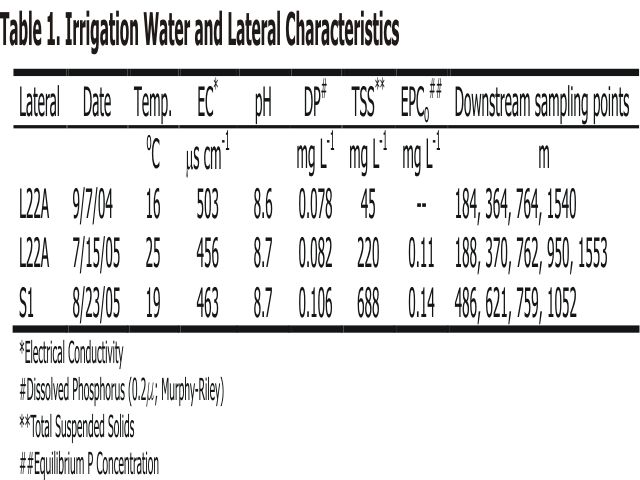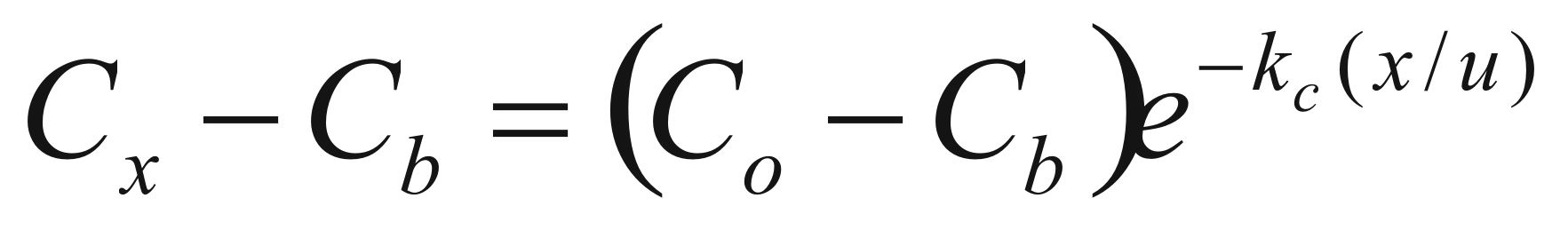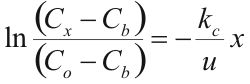Research Design and Methods

 In-stream Phosphorus Injection A solution of K2HPO4 ( 2000 mg P L-1) and KBr (50,000 mg Br L-1) was injected at a constant rate into Lateral 22A (L22A) and S1 Coulee (S1). After 1.5 hr of injection, (previously determined as the time required for Br concentrations to plateau) water samples were collected at 1 upstream location and 4 to 5 down stream locations every 10 to 20 min for 1.5 hr. Samples were immediately filtered, returned to the lab, and analyzed for dissolved P and Br. Select samples were kept and analyzed for pH, electrical conductivity (EC), and total suspended solids (TSS). Automated samplers set up at a minimum of two down-stream locations were used to confirm plateau concentrations. Bottom sediments were collected for determination of equilibrium P concentration (EPCo).Calculation of P Uptake Length Phosphorus uptake length is a measure of the average distance an ortho-phosphate molecule travels prior to biotic or abiotic uptake. Phosphorus uptake length was calculated based on the longitudinal decrease in DP downstream from the steady-state in-stream P injection. A simplified model linking P transformations to down-stream transport during steady-state P injection can be written as:Equation 1 where Cx is the P concentration (mg L-1) at some distance x (m) down stream, Cb is the background P concentration, Co is the P concentration at x=0, kc is the uptake rate coefficient (s-1), and u is the stream velocity (m s-1). Equation 1 can be re-arranged to yield:Equation 2 Phosphorus uptake length (Sw) can be calculated as: Sw=u/kc. In accordance with Eq. 2, a plot of ln[(Cx–Cb)/(Co–Cb)] will give a straight line with slope (a) equal to –kc/u, therefore, Sw= –1/a.